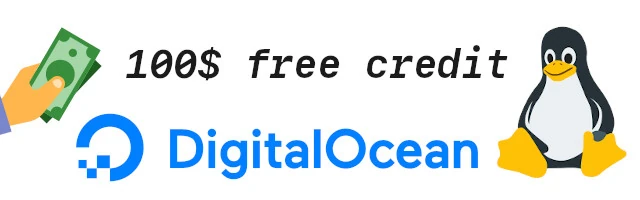# qpdldecode

## Decode a QPDL stream into human readable form.

qpdldecode [options] <qpdl-file

qpdldecode decodes a QPDL stream into human readable form. Only the JBIG compression format (0x13) is handled.

An QPDL stream is the printer language used by the Samsung CLP-300, CLP-600, CLX-3160 and the Xerox Phaser 6110 printers.

These are the options that can appear on the command line.
-d
basename

Basename of .pbm file for saving decompressed planes.

-h

Print hex file offsets.

-o

Print file offsets.

-D level

Set Debug level .

Decode an QPDL stream file created by foo2qpdl.

` 0: \033%-12345X@PJL DEFAULT SERVICEDATE=20070212 2c: @PJL SET USERNAME="Unknown" 49: @PJL SET JOBNAME="testpage.pdf" 6a: @PJL SET COLORMODE=COLOR 84: @PJL SET PAPERTYPE = NORMAL a1: @PJL ENTER LANGUAGE = QPDL bd: RECTYPE 0x0 len=17 res=600, copies=1, papersize=letter(0), w=2550, h=3300 papersource=auto, unk=0, duplex=0:0, unk=0,2, unk=268(0x10c) ce: RECTYPE 0xc len=68(0x44) stripe=0, WB=1248(0x4e0), H=128(0x80), plane=4, comp=0x13, len=56(0x38) magic=0x39abcdef, len=20(0x14), unk=0,0,0,0,0,0, checksum=0x356 DL = 0, D = 0, P = 1, - = 0, XY = 9984 x 6400 L0 = 6400, MX = 0, MY = 0 Order = 0 Options = 72 LRLTWO TPBON 1 stripes, 0 layers, 1 planes 112: RECTYPE 0xc len=68(0x44) stripe=0, WB=1248(0x4e0), H=128(0x80), plane=1, comp=0x13, len=56(0x38) magic=0x39abcdef, len=20(0x14), unk=0,0,0,0,0,0, checksum=0x356 DL = 0, D = 0, P = 1, - = 0, XY = 9984 x 6400 L0 = 6400, MX = 0, MY = 0 Order = 0 Options = 72 LRLTWO TPBON 1 stripes, 0 layers, 1 planes 156: RECTYPE 0xc len=68(0x44) stripe=0, WB=1248(0x4e0), H=128(0x80), plane=2, comp=0x13, len=56(0x38) magic=0x39abcdef, len=20(0x14), unk=0,0,0,0,0,0, checksum=0x356 DL = 0, D = 0, P = 1, - = 0, XY = 9984 x 6400 L0 = 6400, MX = 0, MY = 0 Order = 0 Options = 72 LRLTWO TPBON 1 stripes, 0 layers, 1 planes 19a: RECTYPE 0xc len=68(0x44) stripe=0, WB=1248(0x4e0), H=128(0x80), plane=3, comp=0x13, len=56(0x38) magic=0x39abcdef, len=20(0x14), unk=0,0,0,0,0,0, checksum=0x356 DL = 0, D = 0, P = 1, - = 0, XY = 9984 x 6400 L0 = 6400, MX = 0, MY = 0 Order = 0 Options = 72 LRLTWO TPBON 1 stripes, 0 layers, 1 planes 1de: RECTYPE 0xc len=77488(0x12eb0) stripe=1, WB=1248(0x4e0), H=128(0x80), plane=1, comp=0x13, len=77476(0x12ea4) magic=0x39abcdef, len=77440(0x12e80), unk=2000000,0,0,0,0,0, checksum=0x9326d7 1308e: RECTYPE 0xc len=77680(0x12f70) stripe=1, WB=1248(0x4e0), H=128(0x80), plane=2, comp=0x13, len=77668(0x12f64) magic=0x39abcdef, len=77632(0x12f40), unk=2000000,0,0,0,0,0, checksum=0x9367e5 25ffe: RECTYPE 0xc len=69232(0x10e70) stripe=1, WB=1248(0x4e0), H=128(0x80), plane=3, comp=0x13, len=69220(0x10e64) magic=0x39abcdef, len=69184(0x10e40), unk=2000000,0,0,0,0,0, checksum=0x83938a 36e6e: RECTYPE 0xc len=45616(0xb230) stripe=1, WB=1248(0x4e0), H=128(0x80), plane=4, comp=0x13, len=45604(0xb224) magic=0x39abcdef, len=45568(0xb200), unk=2000000,0,0,0,0,0, checksum=0x58015d 4209e: RECTYPE 0x1 len=3 copies=1 420a1: RECTYPE 0x9 len=0 420a2: \033%-12345X`

/usr/bin/qpdldecode

foo2qpdl-wrapper(1), foo2qpdl(1)

Rick Richardson <rick.richardson@comcast.net>
http://foo2qpdl.rkkda.com/